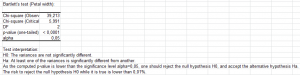# k-sample comparison of variances

k-sample comparison of variance tests (Bartlett and Levene tests) are used to compare several variances. Available in Excel with the XLSTAT software.## What are k-sample comparison of variances tests

Take k samples S1, S2, …, Sk, comprising n1, n2, …, nk observations with variances s1², s2², …, sk². The k-sample comparison of variances will test if at least one of the Si is different from the others.

## k-sample comparison of variances in XLSTAT

XLSTAT offers two parametric tests for the comparison of the variances of k independent samples (k > 2).

### Levene's test

Levene's test can be used to compare two or more variances. This is a two-tailed test for which the null and alternative hypotheses are:

• H0: s1² = s2² = … = sk²
• Ha: There is at least one pair (i, j) such that si² ≠ sj²

The statistic from this test involves absolute deviations at the mean or at the median. The use of the mean is recommended for symmetrical distributions with averagely thick tails. The use of the median is recommended for asymmetric distributions.

The Levene statistic follows a Fisher distribution with k-1 and n1+n2-2 degrees of freedom.

### Bartlett’s homogeneity of variances test

Bartlett's test can be used to compare two or more variances. This test is sensitive to the normality of the data. In other words, if the hypothesis of normality of the data seems fragile, it is better to use Levene's or Fisher's test. On the other hand, Bartlett's test is more powerful if the samples follow a normal distribution.

This also is a two-tailed test which can be used with two or more variances. The hypothesis are similar to the ones in the Levene’s test. Bartlett's statistic follows a Chi² distribution with k-1 degree of freedom.### analyze your data with xlstat

14-day free trial

Included in

Related features